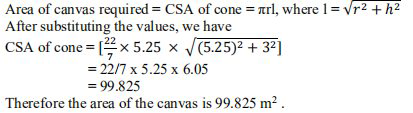# NCERT Solutions Class 9 Mathematics Solutions for Surface Areas and Volumes - Exercise 13.7 in Chapter 13 - Surface Areas and Volumes

Question 9 Surface Areas and Volumes - Exercise 13.7

A heap of wheat is in the form of a cone whose diameter is 10.5 m and height is 3 m. Find its

volume. The heap is to be covered by canvas to protect it from rain. Find the area of the canvas.

(Assume π = 22/7)

Radius (r) of heap = (10.5/2) m = 5.25

Height (h) of heap = 3m

\text { Volume of heap }=1 / 3 \pi \mathrm{r}^{2} \mathrm{h}

= 1/3 x 22/7 x 5.25 x 5.25 x 3

= 86.625

The volume of the heap of wheat is

86.625 \mathrm{m}^{3}

Again,Related Questions

Lido

Courses

Teachers

Book a Demo with us

Syllabus

Maths
CBSE
Maths
ICSE
Science
CBSE

Science
ICSE
English
CBSE
English
ICSE
Coding

Terms & Policies

Selina Question Bank

Maths
Physics
Biology

Allied Question Bank

Chemistry
Connect with us on social media!